# Fe metal sheet

For one product, 5/8 of the metal sheet are consumed, to the second 5/6 of remains. What part of the sheet metal is consumed for both products together?

Correct result:

x =  0.9375

#### Solution: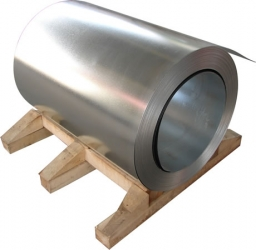We would be pleased if you find an error in the word problem, spelling mistakes, or inaccuracies and send it to us. Thank you!Tips to related online calculators
Need help calculate sum, simplify or multiply fractions? Try our fraction calculator.

## Next similar math problems:

• Evaluate expressionEvaluate expression using BODMAS rule: 1 1/4+1 1/5÷3/5-5/8
• Wood 11Father has 12 1/5 meters long wood. Then I cut the wood into two pieces. One part is 7 3/5 meters long. Calculate the length of the other wood?
• Quotient 3If the quotient of 8/13 and 2 is subtracted from the product of 1 3/4 and 8/21, what is the difference?7 is added to the sum of 4/5 and 6/7
• Length 7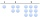Length equals 7/6 inch in width equals 7/9 inches the area is?3 3/4 + 2 3/5 + 5 1/2 Show your solution.
• Numbers divisionWith what number should be divided mixed number 2 3/4 to get 11/12?
• TimeWrite time in minutes rounded to one decimal place: 5 h 28 m 26 s.
• Unknown number 6Determine x if 1/6 of x is equal to 2/5 of the number 24.
• Ratio6 numbers are in the ratio 1:5:1:5:5:5. Their sum is 242. What are the numbers?
• Stock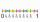Enterprise sold 7/12 of their products to foreign markets and 2/5 of the remainder sold at home. How many % of the products is still in stock?
• Trees3/5 trees are apples, cherries are 1/3. 5 trees are pear. How many is the total number of trees?
• Brackets 2Add parenthesis to make true: 5-2×6-4+2=5
• The ketchup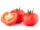If 3 1/4 of tomatoes are needed to make 1 bottle of ketchup. Find the number of tomatoes required to make 4 1/5 bottles
• Length subtractingExpress in mm: 5 3/10 cm - 2/5 mm
• Division of moneyCalculate how many euros have Matthew, Miriam, Lucy, Michael, Janka when together have 2,700 euros and the amounts are at a ratio of 1:5:6:7:8.
• The horses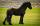A couple of horses consume 88 kg of oats for 14 days. How many oats consumed 7 horses at the same consumption for 6 days?